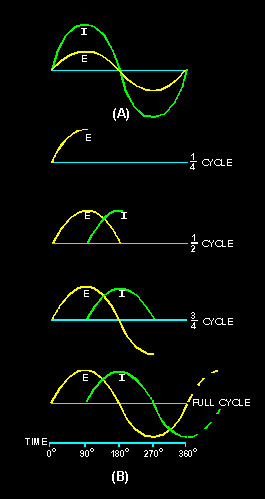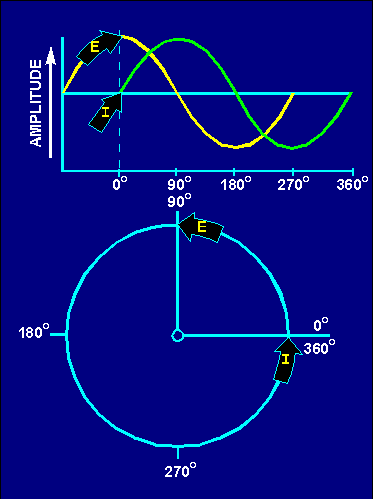INDUCTIVE AND CAPACITIVE REACTANCE phase relationships between current and voltage in an inductor and in a capacitor. State the terms for the opposition an inductor and a capacitor offer to ac Write the formulas for inductive and capacitive reactances. State the effects of a change in frequency on X L and XC. State the effects of a change in inductance on X L and a change in capacitance on XC. ">Custom SearchINDUCTIVE AND CAPACITIVE REACTANCE LEARNING OBJECTIVES Upon completion of this chapter you will be able to: State the effects an inductor has on a change in current and a capacitor has on a change in voltage. State the phase relationships between current and voltage in an inductor and in a capacitor. State the terms for the opposition an inductor and a capacitor offer to ac Write the formulas for inductive and capacitive reactances. State the effects of a change in frequency on X L and XC. State the effects of a change in inductance on X L and a change in capacitance on XC. Write the formula for determining total reactance (X); compute total reactance (X) in a series circuit; and indicate whether the total reactance is capacitive or inductive. State the term given to the total opposition (Z) in an ac circuit. Write the formula for impedance, and calculate the impedance in a series circuit when the values of XC, X L, and R are given. Write the Ohm's law formulas used to determine voltage and current in an ac circuit. Define true power, reactive power, and apparent power; state the unit of measurement for and the formula used to calculate each. State the definition of and write the formula for power factor. Given the power factor and values of X and R in an ac circuit, compute the value of reactance in the circuit, and state the type of reactance that must be connected in the circuit to correct the power factor to unity (1). State the difference between calculating impedance in a series ac circuit and in a parallel ac circuit. INDUCTIVE AND CAPACITIVE REACTANCE You have already learned how inductance and capacitance individually behave in a direct current circuit. In this chapter you will be shown how inductance, capacitance, and resistance affect alternating current. INDUCTANCE AND ALTERNATING CURRENT This might be a good place to recall what you learned about phase in chapter 1. When two things are in step, going through a cycle together, falling together and rising together, they are in phase. When they are out of phase, the angle of lead or lag-the number of electrical degrees by which one of the values leads or lags the other-is a measure of the amount they are out of step. The time it takes the current in an inductor to build up to maximum and to fall to zero is important for another reason. It helps illustrate a very useful characteristic of inductive circuits-the current through the inductor always lags the voltage across the inductor. A circuit having pure resistance (if such a thing were possible) would have the alternating current through it and the voltage across it rising and failing together. This is illustrated in figure 4-1(A),which shows the sine waves for current and voltage in a purely resistive circuit having an ac source. The current and voltage do not have the same amplitude, but they are in phase. In the case of a circuit having inductance, the opposing force of the counter emf would be enough to keep the current from remaining in phase with the applied voltage. You learned that in a dc circuit containing pure inductance the current took time to rise to maximum even though the full applied voltage was immediately at maximum. Figure 4-1(B) shows the wave forms for a purely inductive ac circuit in steps of quarter-cycles. Figure 4-1. - Voltage and current waveforms in an inductive circuit.With an ac voltage, in the first quarter-cycle (0° to 90°) the applied ac voltage is continually increasing. If there was no inductance in the circuit, the current would also increase during this first quarter-cycle. You know this circuit does have inductance. Since inductance opposes any change in current flow, no current flows during the first quarter-cycle. In the next quarter-cycle (90° to 180°) the voltage decreases back to zero; current begins to flow in the circuit and reaches a maximum value at the same instant the voltage reaches zero. The applied voltage now begins to build up to maximum in the other direction, to be followed by the resulting current. When the voltage again reaches its maximum at the end of the third quarter-cycle (270°) all values are exactly opposite to what they were during the first half-cycle. The applied voltage leads the resulting current by one quarter-cycle or 90 degrees. To complete the full 360° cycle of the voltage, the voltage again decreases to zero and the current builds to a maximum value. You must not get the idea that any of these values stops cold at a particular instant. Until the applied voltage is removed, both current and voltage are always changing in amplitude and direction. As you know the sine wave can be compared to a circle. Just as you mark off a circle into 360 degrees, you can mark off the time of one cycle of a sine wave into 360 electrical degrees. This relationship is shown in figure 4-2. By referring to this figure you can see why the current is said to lag the voltage, in a purely inductive circuit, by 90 degrees. Furthermore, by referring to figures 4-2 and 4-1(A) you can see why the current and voltage are said to be in phase in a purely resistive circuit. In a circuit having both resistance and inductance then, as you would expect, the current lags the voltage by an amount somewhere between 0 and 90 degrees. Figure 4-2. - Comparison of sine wave and circle in an inductive circuit.A simple memory aid to help you remember the relationship of voltage and current in an inductive circuit is the word ELI. Since E is the symbol for voltage, L is the symbol for inductance, and I is the symbol for current; the word ELI demonstrates that current comes after (Lags) voltage in an inductor. Q.1 What effect does an inductor have on a change in current? Q.2 What is the phase relationship between current and voltage in an inductor?Integrated Publishing, Inc.